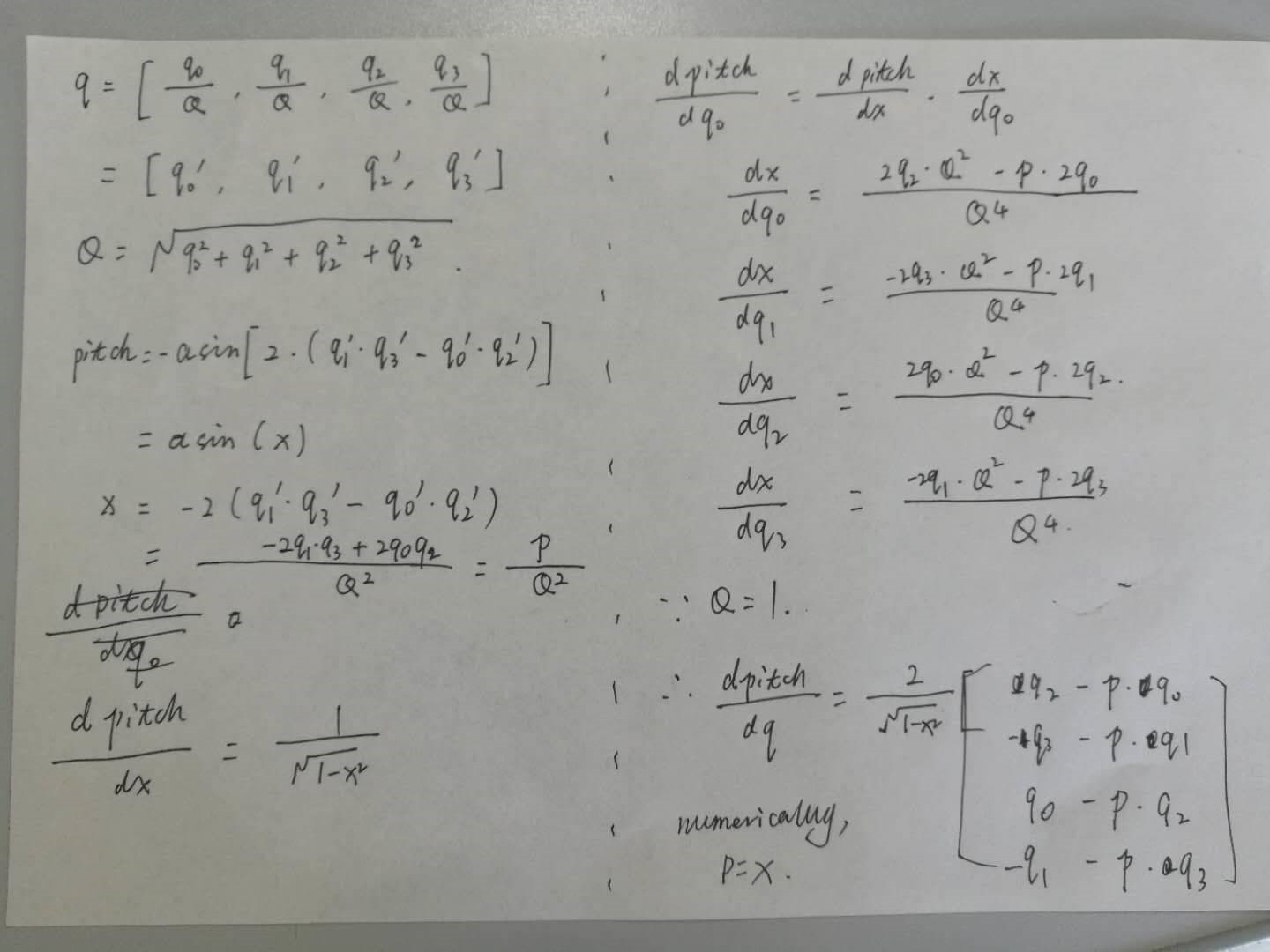# Wheel angle in EKF

Hello

I intend to use IMU300ZI in our omnidirectional robot (can turn all 4 wheels into arbitrary but the same direction). The robot has speed encoder and wheel angle encoder so I want to use this info in EKF. As a base code I use your INS example project.
I studied your code and think that the best place for wheel angle incorporation is in function `Update_PseudoMeasurement()` in UpdateFunctions.c. Instead of using `frontVelMea` variable as scalar I would make it vector the values of wich would be set based on current wheel angle, wheel speed and `gKalmanFilter.ppsEulerAngles[YAW]`. I would also set `gAlgorithm.velocityAlwaysAlongBodyX=FALSE`.

Odometer data would be received over SPI.
Do you think this is correct solution or is there anything important I forgot about which could cause failure?

Thank you
Regards
Pavel

Hello,

I think you are right. Just a reminder that the measured velocity in the body frame is no longer [frontVelMean, 0, 0] now. You may also rename Update_PseudoMeasurement() to something else because you are using actual measurements instead of pseudo ones.

Thank you very much for the reply.
I'd like to ask one more question about function `_GenerateObservationJacobian_AHRS` in UpdateFunctions.c.

There is following comment for `uTheta`

``````/* Pitch (including modifications for |q| = 1 constraint)
*   mu = 2*( q(1)*q(3) - q(2)*q(4) );
*   % 2/sqrt(1-mu*mu) * [q(3); -q(4); q(1); -q(2)]
*   2/sqrt(1-mu*mu) * [q(3) - mu*q(1);
*                      -q(4) - mu*q(2);
*                     q(1)- mu*q(3);
*                     -q(2)- mu*q(4)]
*/
``````

I know how to get this line

``````% 2/sqrt(1-mu*mu) * [q(3); -q(4); q(1); -q(2)]
``````

but don't know how you came to this

``````2/sqrt(1-mu*mu) * [q(3) - mu*q(1);
*                      -q(4) - mu*q(2);
*                     q(1)- mu*q(3);
*                     -q(2)- mu*q(4)]
``````

which is most probably mentioned constraint for |q| = 1.

Could you please shed more light on it? Your code awesome and great practical EKF tutorial.

Thank you
regards
Pavel

@paha

I found the derivation. I have used numerical differential of quaternion as reference to compare the two formula. The one with constraint did give better accuracy when pitch and roll are not close to 0.I got it now.
Thank you very much
Regards
Pavel